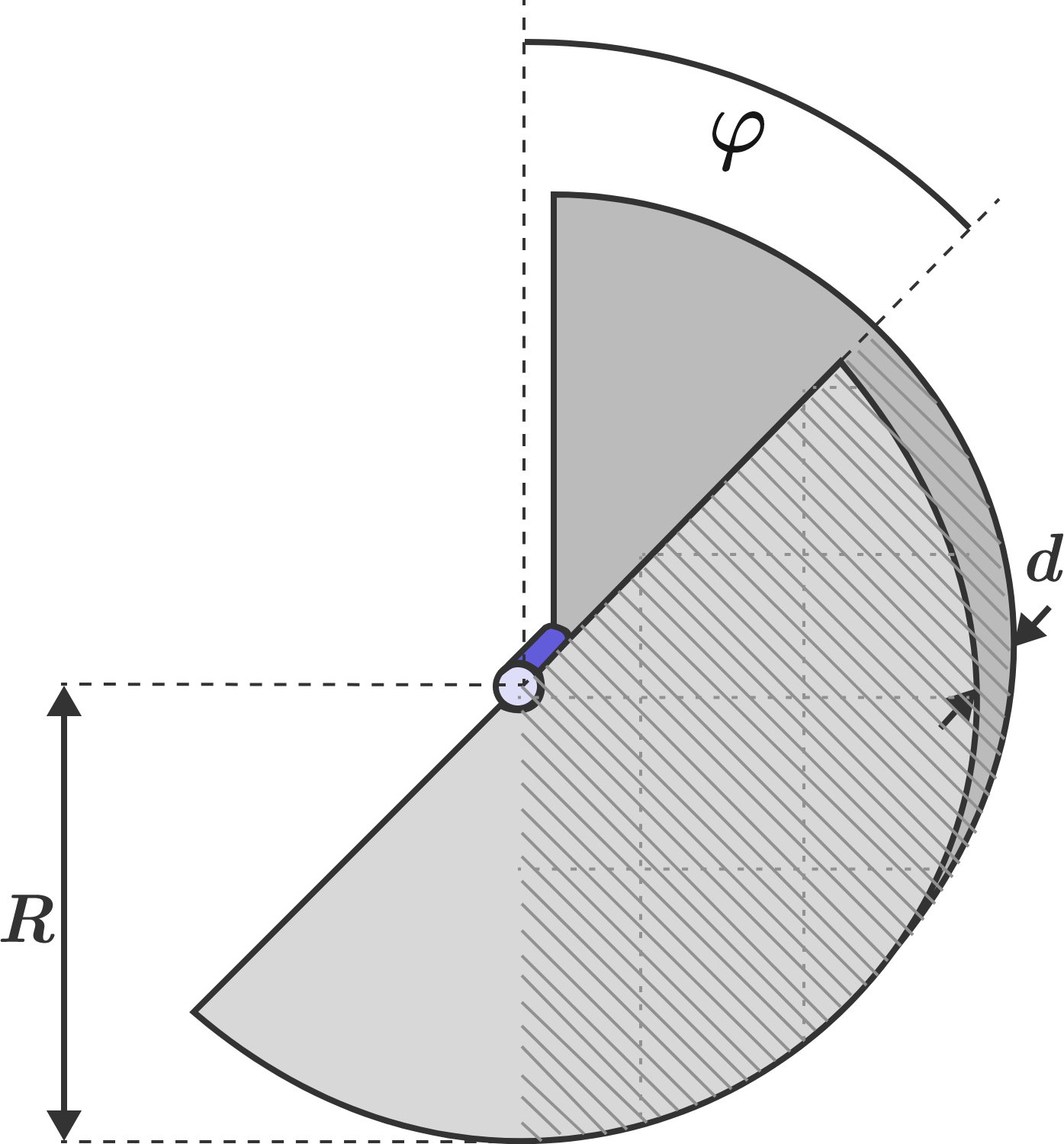# Rotary variable capacitorA variable capacitor consists of two metal semicircles of radius $R$ with vertical separation $d.$ The capacitor is charged when $\varphi=0,$ and is then disconnected from the voltage source. The discs are then rotated through the angle $\varphi = 90^\circ.$

How does the rotation change the energy that's stored in the capacitor?

×